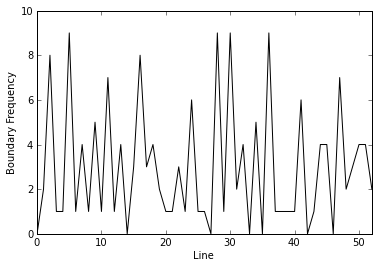In :
%pylab inline

Welcome to pylab, a matplotlib-based Python environment [backend: module://IPython.zmq.pylab.backend_inline].


# An initial study of topical poetry segmentation¶

This work investigates topical segmentation of poetry to better understand its interpretation humans. Nine segmentations of the poem titled Kubla Khan (Coleridge, 1816) were collected; a small number, but enough to inform a future larger study and to obtain feedback upon the methodologies used.

Chris Fournier. 2013. An initial study of topical poetry segmentation. Proceedings of the Second Workshop on Computational Linguistics for Literature, pp. 47-51. Association for Computational Linguistics, Stroudsburg, PA, USA.

In :
# Import required libraries
import os
import csv
import segeval as se
import numpy as np
import matplotlib.pyplot as plt
import itertools as it
from collections import defaultdict
from decimal import Decimal
from hcluster import linkage, dendrogram, fcluster


Nine Master Tukers were recruited using from the United States and were asked to segment the poem into topically contiguous segments at the line level. They were also asked to produce one-sentence summaries of each segment.

Data

• The segmentations themselves were saved within the file kubla_khan_fournier_2013.json
• The principle researcher read these summaries and attempted to label the type of segments that the Turkers produced which is saved as labels.csv.

To later perform comparisons in an order that hcluster expects, an ordered list of coders is defined herein named coders.

In :
# Document to analyse
item_name = u'kublakhan'
number_of_lines = 54

# Ordered list of coders (and numeric list of coders) used to relate
# numbered cluster coders to other graphs
coders = ['AWRAXV1RIYR0M', 'A23S6QOSZH9TMT', 'A21IFZJ0EDKM4E', 'AO3XB5I5QNNUI', 'A3RLCGRXA34GC0', 'A21SF3IKIZB0VN', 'APXNY64HXO08K', 'AM155T4U3RE1A', 'A2YBGZ2H2KSO5T']
labels = ['%i' % i for i in range(0, len(coders))]

filepath = os.path.join('data', 'kubla_khan_fournier_2013.json')
dataset = se.input_linear_mass_json(filepath)

segment_labels = dict()
filepath = os.path.join('data', 'kubla_khan_fournier_2013', 'labels.csv')
with open(filepath) as csv_file:
segment_labels[row] = [item.strip() for item in row[1:]]


## Compute descriptive statistics¶

Two descriptive statistics are used to analyse the codings that the coders produced:

• Boundary Similarity (B) to analyse the boundaries placed by segmenters; and
• Jaccard Similarity (J) of the labels describing the segments (where the labels for each segment are placed upon each line before computing similarity).

Boundary SImilarity is described in:

Chris Fournier. 2013. Evaluating Text Segmentation using Boundary Edit Distance. Proceedings of the 51st Annual Meeting of the Association for Computational Linguistics. Association for Computational Linguistics, Stroudsburg, PA, USA.

In :
# Compute boundaries
boundaries = dict([(key, len(mass) - 1) for key, mass in dataset[item_name].items()])
coder_boundaries = [boundaries[coder] for coder in coders]

# Compute similarities (1-B)
similarities = se.boundary_similarity(dataset, one_minus=True)

In :
# Expand segment labels using the mass of each segment to create
# a one to one mapping between line and segment label
expanded_segment_labels = defaultdict(list)
for coder in coders:
masses = dataset[item_name][coder]
coder_segment_labels = segment_labels[coder]
expanded_segment = list()
for mass, coder_segment_label in zip(masses, coder_segment_labels):
expanded_segment.extend(list([coder_segment_label]) * mass)
expanded_segment_labels[coder] = expanded_segment

# Define label similarity function
def jaccard(a, b):
return float(len(a & b)) / float(len(a | b))

# Compute overall label Jaccard similarities per position
total_similarities = list()
row_similarities = list()
for i in xrange(0, number_of_lines):
parts = list()
for coder in coders:
parts.append(set(expanded_segment_labels[coder][i].split('/')))
part_combinations = it.combinations(parts, 2)
position_similarities = [jaccard(a, b) for a, b in part_combinations]
total_similarities.extend(position_similarities)
row_similarities.append(position_similarities)


## Define helper functions¶

Functions that aid in graphing.

In :
def autolabel(rects, rotation=0, xpad=0):
# attach some text labels
for rect in rects:
height = rect.get_height()
ha='center', va='bottom', rotation=rotation)


## Overall analysis¶

In :
similarity_values = [float(value) for value in similarities.values()]
mean_b = np.mean(similarity_values)
std_b  = np.std(similarity_values)

mean_j = np.mean(total_similarities)
std_j = np.std(total_similarities)

print 'Mean B \t\t {0:.4f} +/- {1:.4f}, n={2}'.format(mean_b, std_b, len(similarity_values))
print 'Mean J \t\t {0:.4f} +/- {1:.4f}, n={2}'.format(mean_j, std_j, len(total_similarities))
print 'Fleiss\' Pi \t {0:.4f}'.format(se.fleiss_pi_linear(dataset))

Mean B 		 0.5375 +/- 0.1158, n=36
Mean J 		 0.5330 +/- 0.4567, n=1944
Fleiss' Pi 	 0.3789


## Subset analysis¶

The overall statistics show that the 9 coders have low agreement regardless of the metric used.

Cluster segmentations by boundary similarity

Hypothesis: Subsets of the coders may agree better with eachother.

To explore this hypothesis, the similarities of the boundaries placed within each segmentation (1-B) were used as a distance function to perform hierarchical agglomerative clustering. Each cluster can then be analyzed.

In :
# Order distances for clustering
coder_combinations = [list(a) for a in it.combinations(coders, 2)]
for coder_combination in coder_combinations:
coder_combinations.reverse()
keys = list()
for a in coder_combinations:
a = list(a)
key = ','.join([item_name] + a)
if key not in similarities:
a.reverse()
key = ','.join([item_name] + a)
keys.append(key)
distances = [similarities[key] for key in keys]

In :
# Cluster
dendro = dendrogram(aglomerative_clusters, labels=labels)
plt.ylabel('Mean Distance (1-B)')
plt.xlabel('Coder')
plt.show(dendro)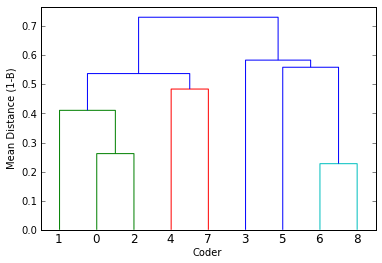Compute statistics for each cluster

Given the clusters produced above, let's calculate statistics for each cluster.

In :
cluster_members = {
'0,2' : [coders, coders],
'1,0,2' : [coders, coders, coders],
'4,7' : [coders, coders],
'1,0,2,4,7' : [coders, coders, coders, coders, coders],
'6,8' : [coders, coders],
'5,6,8' : [coders, coders, coders],
'3,5,6,8' : [coders, coders, coders, coders]
}

cluster_pi = dict()
cluster_b  = dict()
cluster_j  = dict()
for cluster, members in cluster_members.items():
data = {coder : dataset[item_name][coder] for coder in members}
dataset_subset = se.Dataset({item_name : data})
cluster_b[cluster]  = [float(value) for value in se.boundary_similarity(dataset_subset, n_t=2).values()]
cluster_pi[cluster] = float(se.fleiss_pi_linear(dataset_subset, n_t=2))
position_j = list()
for i in xrange(0, number_of_lines):
parts = list()
for coder in members:
parts.append(set(expanded_segment_labels[coder][i].split('/')))
part_combinations = it.combinations(parts, 2)
position_similarities = [jaccard(a, b) for a, b in part_combinations]
position_j.extend(position_similarities)
cluster_j[cluster] = position_j

In :
print 'Cluster\t\tPi\tB\t\t\tJ'
for cluster in cluster_members.keys():
print '{0}\t{1:.4f}\t{2:.4f} +/- {3:.4f}, n={4}\t{5:.4f} +/- {6:.4f}, n={7}'.format(cluster if len(cluster) > 7 else cluster+'\t',
np.mean(cluster_pi[cluster]),
np.mean(cluster_b[cluster]),
np.std(cluster_b[cluster]),
len(cluster_b[cluster]),
np.mean(cluster_j[cluster]),
np.std(cluster_j[cluster]),
len(cluster_j[cluster]))

Cluster		Pi	B			J
4,7		0.3704	0.5161 +/- 0.0000, n=1	0.4907 +/- 0.4953, n=54
0,2		0.6946	0.7381 +/- 0.0000, n=1	0.4599 +/- 0.4385, n=54
6,8		0.7625	0.7727 +/- 0.0000, n=1	0.6852 +/- 0.4644, n=54
1,0,2		0.5520	0.6400 +/- 0.0694, n=3	0.5082 +/- 0.4524, n=162
1,0,2,4,7	0.4474	0.5623 +/- 0.0792, n=10	0.5120 +/- 0.4672, n=540
3,5,6,8		0.4764	0.5187 +/- 0.1239, n=6	0.5926 +/- 0.4245, n=324
5,6,8		0.5389	0.5909 +/- 0.1372, n=3	0.5802 +/- 0.4320, n=162


Plot mean similarities per cluster

The mean boundary similarity (B) and mean Jaccard label similarity (J), with standard deviation, is shown below.

In :
y = list()
y2 = list()
y2err = list()
y3 = list()
y3err = list()

for cluster in cluster_members.keys():
y.append(float(cluster_pi[cluster]))
y2.append(np.mean(cluster_b[cluster]))
y2err.append(np.std(cluster_b[cluster]))
y3.append(np.mean(cluster_j[cluster]))
y3err.append(np.std(cluster_j[cluster]))

ind = np.arange(len(cluster_members))  # the x locations for the groups
width = 0.26       # the width of the bars

fig = plt.figure()
rects1 = ax.bar(ind, y, width, color='0.25', ecolor='k')
rects2 = ax.bar(ind+width, y2, width, yerr=y2err, color='0.5', ecolor='k')
rects3 = ax.bar(ind+width*2, y3, width, yerr=y3err, color='0.75', ecolor='k')

ax.set_ylabel('Cluster similarity')
ax.set_xticks(ind + ((width * 3) / 2))
ax.set_xticklabels(labels)
ax.set_xlim([-0.25,6.95])
ax.set_ylim([0,1])

ax.legend( (rects1, rects2, rects3), ('$\kappa_{\mathrm{B}}$', 'E(B)', 'E(J)') )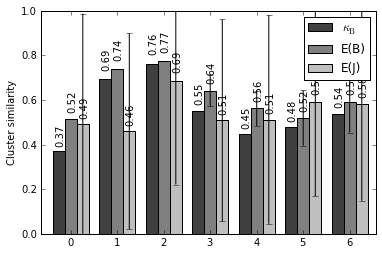## Coder analysis¶

Having looked at subsets of coders, it would be informative to also analyze coder behaviour overall.

Plot boundary placement frequency

To visualize coder behaviour, this plot indicates the frequency at which various coders placed boundaries in this document.

In :
# Plot boundaries per coder
y = coder_boundaries
x = np.arange(len(y))

# Set up
width = 0.75
fig = plt.figure()

# Plot
rects = ax.bar(x, y, width, color='0.75')

ax.set_xticks(x + (width / 2))
ax.set_xticklabels([str(val) for val in labels])

# Draw mean lines
xmin, xmax, ymean, ystd = -0.25, len(labels), np.mean(y), np.std(y)
ax.plot([xmin, xmax], [ymean] * 2, color='k') # Draw mean
ax.plot([xmin, xmax], [ymean + ystd] * 2, color='0.5') # Draw +std
ax.plot([xmin, xmax], [ymean - ystd] * 2, color='0.5') # Draw -std

format_str='%d'
fnc_value=int
for rect in rects:
height = rect.get_height()
ax.text(rect.get_x() + rect.get_width() / 2., 1.05 * height, format_str%fnc_value(height), ha='center', va='bottom')

# Format
ax.set_xlim([-0.25, 9])
ax.set_ylim([0, 30])
ax.set_xlabel('Coder')
ax.set_ylabel('Boundaries placed (quantity)')
plt.show()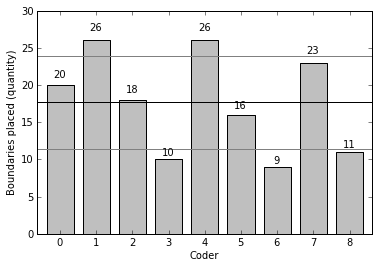Plot coder label similarity per line

To visualize the areas of the poem which had the greatest agreement in terms of topic segment type, the Jaccard similarity per position between all coders was plotted.

In :
# Create heat map
y_sim = list()
y_sim_err = list()
for row_similarity in row_similarities:
y_sim.append(np.mean(row_similarity))
y_sim_err.append(np.std(row_similarity))

# Plot mean label similarity
labels = ['$%i$' % i for i in range(0, number_of_lines)]

y = list(y_sim)
x = range(0, number_of_lines)
plt.errorbar(x, y, color='k', )

xlim([0, number_of_lines - 1])
ylim([0, 1.05])

plt.ylabel('Mean Label Jaccard Similarity')
plt.xlabel('Line')

plt.show()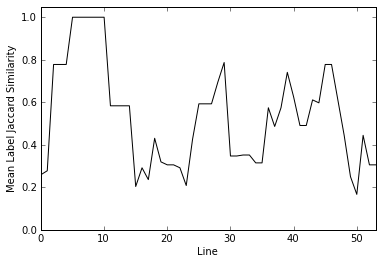Plot coder boundary frequency per line

To visualize the areas of the poem which had the greatest number of boundaries placed by all coders, the boundary frequency per position for all coders was plotted.

In :
position_frequency =  * (sum(dataset['kublakhan'].values()) - 1)

for segmentation in dataset['kublakhan'].values():
position = 0
for segment in segmentation[0:-1]:
position += segment
position_frequency[position] += 1

position_boundary_sim = [float(value) / 9 for value in position_frequency]

In :
# Create heat map
y = position_frequency

# Plot mean label similarity
labels = ['$%i$' % i for i in range(0, number_of_lines)]

x = range(0, number_of_lines - 1)
plt.errorbar(x, y, color='k', )

xlim([0, 52.0])
ylim([0, 10])

plt.ylabel('Boundary Frequency')
plt.xlabel('Line')

plt.show()